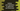# Python program to print all alphabets from A to Z in uppercase and lowercase## Python program to print all alphabets from a to z in both lowercase and uppercase:

In this post, we will learn how to print all alphabets from a to z in both uppercase and lowercase. The easiest way to solve this is by running a for loop on the alphabets .

### By using a for loop:

We can use string.ascii_lowercase and string.ascii_uppercase to get all uppercase and lowercase characters in one string. Then, we can use a for loop to iterate through the characters.

Below is the complete program:

``````import string

for ch in string.ascii_lowercase:
print(ch, end='')
print()

for ch in string.ascii_uppercase:
print(ch, end='')
print()``````

In this program,

• We are importing string because we want to use ascii_lowercase or ascii_uppercase.
• ascii_lowercase returns a string with all the alphabets in uppercase or lowercase.
• We are running a for loop to iterate over the characters and printing the character values.
• After the for loops, it is using print() to print a new line.

If you run this program, it will print the below output:

``````abcdefghijklmnopqrstuvwxyz
ABCDEFGHIJKLMNOPQRSTUVWXYZ``````

### By using chr():

Using chr(), we can convert the ASCII value to character. 97 is the ASCII value of a and 123 is the ASCII value of z. We can loop over the integers from 97 to 123 and using chr(), we can print the alphabets.

Below is the complete program:

``````for no in range(97, 123):
print(chr(no), end='')
print()

for no in range(65, 91):
print(chr(no), end='')
print()``````

It will print the same output.

Here,

• The first for loop prints all chr values from 97 to 123, i.e. all lower case characters
• Similarly, the second for loop prints all chr values from 65 to 91 i.e. all upper case characters.